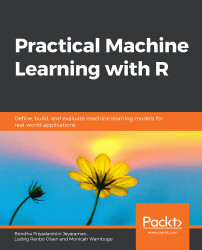•#### Practical Machine Learning with R#### Overview of this book

With huge amounts of data being generated every moment, businesses need applications that apply complex mathematical calculations to data repeatedly and at speed. With machine learning techniques and R, you can easily develop these kinds of applications in an efficient way. Practical Machine Learning with R begins by helping you grasp the basics of machine learning methods, while also highlighting how and why they work. You will understand how to get these algorithms to work in practice, rather than focusing on mathematical derivations. As you progress from one chapter to another, you will gain hands-on experience of building a machine learning solution in R. Next, using R packages such as rpart, random forest, and multiple imputation by chained equations (MICE), you will learn to implement algorithms including neural net classifier, decision trees, and linear and non-linear regression. As you progress through the book, you’ll delve into various machine learning techniques for both supervised and unsupervised learning approaches. In addition to this, you’ll gain insights into partitioning the datasets and mechanisms to evaluate the results from each model and be able to compare them. By the end of this book, you will have gained expertise in solving your business problems, starting by forming a good problem statement, selecting the most appropriate model to solve your problem, and then ensuring that you do not overtrain it.Free Chapter
An Introduction to Machine LearningData Cleaning and Pre-processingFeature EngineeringIntroduction to neuralnet and Evaluation MethodsLinear and Logistic Regression ModelsUnsupervised LearningAppendix## Handling Categorical Variables

Categorical variables are a list of string values or numeric values for an attribute. For instance, gender can be "Male" or "Female". There are two types of categories: nominal and ordinal. In nominal categorical data, there is no ordering among the values in that attribute. This is the case with gender values. Ordinal categories have some order within the set of values. For instance, for temperature "Low," "Medium," and "High" have an order.

• Label Encoding: String literals needs to be converted to numeric values, where "Male" can take value 1 and "Female" can take value 2. This is called integer encoding or label encoding. The integer values have a natural ordering so this may be suitable in cases dealing with categorical data, which is ordinal.
• One-Hot Encoding: For nominal categories, label encoding is not suitable as the natural order of the numbers may be learned by the machine...